# NCERT Solutions for Class 9 Science Motion part 1## myCBSEguide App

CBSE, NCERT, JEE Main, NEET-UG, NDA, Exam Papers, Question Bank, NCERT Solutions, Exemplars, Revision Notes, Free Videos, MCQ Tests & more.

NCERT solutions for Science Motion

## NCERT Class 9 Science Chapter wise Solutions

• 01 –  Matter in Our Surroundings
• 02 – Is Mattter Around us Pure
• 03 – Atoms and Molecules
• 04 – Structure of the Atom
• 05 – The Fundamental Unit of Life
• 06 – Tissues
• 07 – Diversity in Living Organisms
• 08 – Motion
• 09 – Force and Laws of Motion
• 10 – Gravitation
• 11 – Word and Energy
• 12 – Sound
• 13 – Why Do We Fall Ill
• 14 – Natural Resources
• 15 – Improvement in Food Resources

## NCERT Solutions for Class 9 Science Motion part 1

### 1. An object has moved through a distance. Can it have zero displacement? If yes, support your answer with an example.

Ans. Yes, if an object has moved through a distance it can have zero displacement because displacement of an object is the actual change in its position when it moves from one position to the other. So if an object travels from point A to B and then returns back to point A again, the total displacement is zero.

### 2. A farmer moves along the boundary of a square field of side 10 m in 40 s. What will be the magnitude of displacement of the farmer at the end of 2 minutes 20 seconds?

Ans. Distance covered by farmer in 40 seconds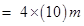= 40 m

Speed of the farmer = distance/time = 40m/40s = 1 m/s.

Total time given in the question = 2min 20seconds = 60+60+20 =140 seconds

Since he completes 1round of the field in 40seconds so in he will complete 3rounds in 120seconds (2mins) or 120m distance is covered in 2minutes. In another 20seconds will cover another 20m so total distance covered in 2min20sec = 120 +20 =140m.

Displacement =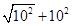200 = 10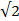m (as per diagram)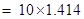= 14.14 m.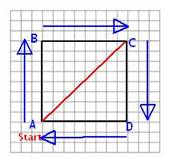### 3. Which of the following is true for displacement?

(a) It cannot be zero.

(b) Its magnitude is greater than the distance travelled by the object.

Ans. Both (a) as well as (b) are false with respect to concept of displacement.

NCERT Solutions for Class 9 Science Motion part 1

### 1. Distinguish between speed and velocity.

Ans. Speed of a body is the distance travelled by it per unit time while velocity is displacement per unit time of the body during movement.

### 2. Under what condition(s) is the magnitude of average velocity of an object equal to its average speed?

Ans. If distance travelled by an object is equal to its displacement then the magnitude of average velocity of an object will be equal to its average speed.

### 3. What does the odometer of an automobile measure?

Ans. The odometer of an automobile measures the distance covered by that automobile.

### 4. What does the path of an object look like when it is in uniform motion?

Ans. Graphically the path of an object will be linear i.e. look like a straight line when it is in uniform motion.

NCERT Solutions for Class 9 Science Motion part 1

### 5. During an experiment, a signal from a spaceship reached the ground station in five minutes. What was the distance of the spaceship from the ground station? The signal travels at the speed of light, that is,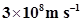.

Ans.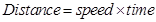=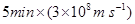=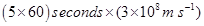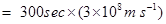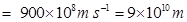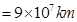NCERT Solutions for Class 9 Science Motion part 1

### 1. When will you say a body is in

(i) uniform acceleration?

(ii) non-uniform acceleration?

Ans. (i) uniform acceleration: When an object travels in a straight line and its velocity changes by equal amount in equal intervals of time, it is said to have uniform acceleration.

(ii) non uniform acceleration: It is also called variable acceleration. When the velocity of an object changes by unequal amounts in equal intervals of time, it is said to have non uniform acceleration.

### 2. A bus decreases its speed from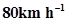to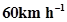in 5 s. Find the acceleration of the bus.

Ans. Initial speed of bus (u) =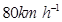=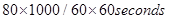=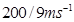=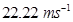final speed of bus (v)=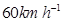=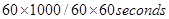=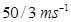=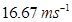time (t) = 5 s

acceleration (a) = ( v – u) /t = (16.67 – 22.22)/5 = -5.55/5 =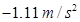### 3. A train starting from a railway station and moving with uniform acceleration attains a speed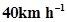in 10 minutes. Find its acceleration.

Ans. Since the train starts from rest(railway station) = u = zero

Final velocity of train =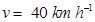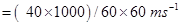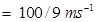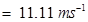time (t) = 10 min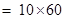= 600 seconds

Since a = (v – u )/t =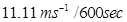=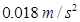NCERT Solutions for Class 9 Science Motion part 1

### 1. What is the nature of the distance-time graphs for uniform and non-uniform motion of an object?

Ans. If an object has a uniform motion then the nature of distance time graph will be linear i.e. it would a straight line and if it has non uniform motion then the nature of distance time graph is a curved line.

### 2. What can you say about the motion of an object whose distance-time graph is a straight line parallel to the time axis?

Ans. If the object’s distance time graph is a straight line parallel to the time axis indicates that with increasing time the distance of that object is not increasing hence the object is at rest i.e. not moving.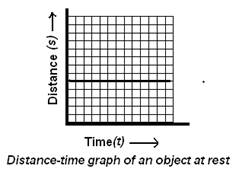NCERT Solutions for Class 9 Science Motion part 1

### 3. What can you say about the motion of an object if its speed time graph is a straight line parallel to the time axis?

Ans. Such a graph indicates that the object is travelling with uniform velocity.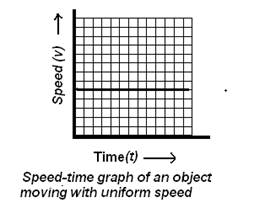### 4. What is the quantity which is measured by the area occupied below the velocity-time graph?

Ans. The area occupied below the velocity-time graph measures the distance moved by any object.

NCERT Solutions for Class 9 Science Motion part 1

### 1. A bus starting from rest moves with a uniform acceleration of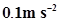for 2 minutes. Find (a)the speed acquired, (b) the distance travelled.

Ans. (a) u=o,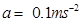, t= 2min = 120 seconds.

v=u+at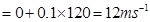so (a) speed acquired =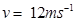(b)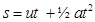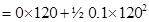= 720 m.

NCERT Solutions for Class 9 Science Motion part 1

### 2. A train is travelling at a speed of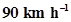. Brakes are applied so as to produce a uniform acceleration of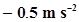. Find how far the train will go before it is brought to rest.

Ans.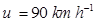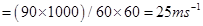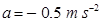, v =0(train is brought to rest)

v= u+at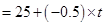0 =25 – 0.5 x

0.5t = 25, or t = 25/0.5 = 50seconds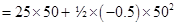= 1250 – 625 = 625m

### 3. A trolley, while going down an inclined plane, has an acceleration of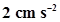. What will be its velocity 3 s after the start?

Ans.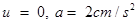, t= 3s

v= u +at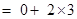= 6 cm/s

### 4. A racing car has a uniform acceleration of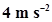. What distance will it cover in 10 s after start?

Ans. u = 0,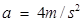, t= 10 s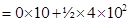= 200 m

NCERT Solutions for Class 9 Science Motion part 1

### 5. A stone is thrown in a vertically upward direction with a velocity of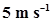. If the acceleration of the stone during its motion is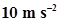in the downward direction, what will be the height attained by the stone and how much time will it take to reach there?

Ans.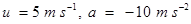v = 0 (since at maximum height its velocity will be zero)

v = u + at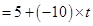0 = 5 – 10t

10t = 5, or, t = 5/10 =0.5second.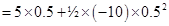= 2.5 – 1.25 = 1.25m

## NCERT Solutions for Class 9 Science

NCERT Solutions Class 9 Science PDF (Download) Free from myCBSEguide app and myCBSEguide website. Ncert solution class 9 Science includes text book solutions .NCERT Solutions for CBSE Class 9 Science have total 15 chapters. 9 Science NCERT Solutions in PDF for free Download on our website. Ncert Science class 9 solutions PDF and Science ncert class 9 PDF solutions with latest modifications and as per the latest CBSE syllabus are only available in myCBSEguide.

## CBSE app for Class 9

To download NCERT Solutions for class 9 Science, Computer Science, Home Science,Hindi ,English, Maths Social Science do check myCBSEguide app or website. myCBSEguide provides sample papers with solution, test papers for chapter-wise practice, NCERT solutions, NCERT Exemplar solutions, quick revision notes for ready reference, CBSE guess papers and CBSE important question papers. Sample Paper all are made available through the best app for CBSE students and myCBSEguide website.### 1 thought on “NCERT Solutions for Class 9 Science Motion part 1”

1. The answers is right but it is a very hard method to solve any question
This methods confused the child that what is wrong and what is right?